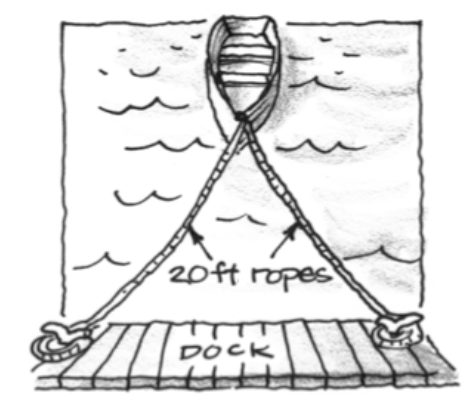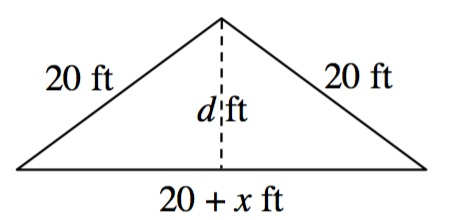### Home > APCALC > Chapter 12 > Lesson 12.3.2 > Problem12-107

12-107.

A rowboat is tethered from its bow to a dock by two ropes, each $20$ feet long. The ropes are tied to the dock at points $20$ feet apart.

The boat’s owner unties one of the ropes from the dock, and brings the boat toward the dock by walking along it away from the other tether point at a rate of $4$ ft/sec. How fast is the boat moving toward the dock when the boat’s owner has walked $10$ feet?Draw a diagram where the $d =$ distance from the dock, which is the height of an isosceles triangle.
Let $x =$ distance the owner has walked.When the owner has walked $10$ ft, by the Pythagorean Theorem, $d = \sqrt{175}$ .

$d^2+\Big(\frac{20+x}{2}\Big)^2=20^2$

$2dd^\prime+\Big(\frac{20+x}{2}\Big)x^\prime=0$

Substitute in the known values and solve for $d′$.